# The Geometric Viewpoint

geometric and topological excursions by and for undergraduates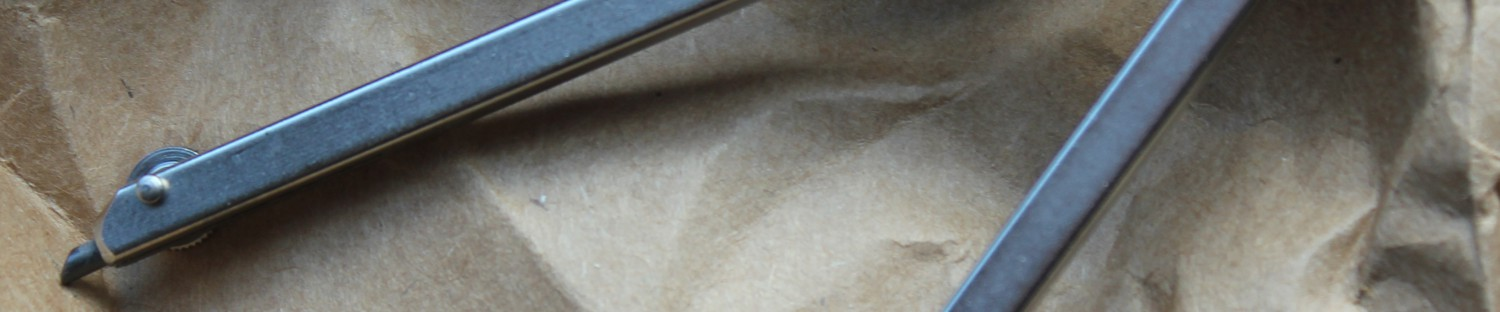## Completeness on an Incomplete Sphere.

I used to think I had a fairly good understanding of distance. If asked to find the distance between two points, I mentally connect them with a line segment and then find its length using the Pythagorean Theorem. Though I knew how to create fancy mathematical spaces, when it came to the real world I had my real sense of distance: straight lines connecting points.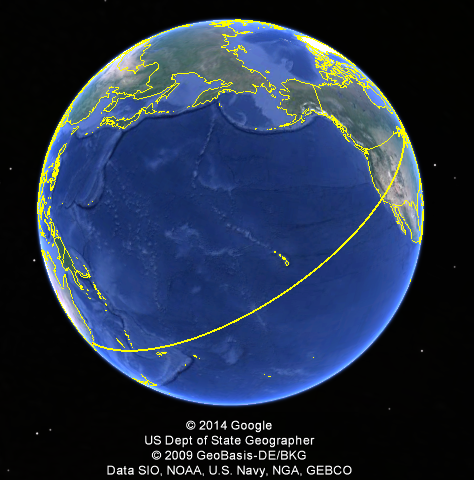Figure 1: When measuring the distance between cities, it doesn’t make sense to find the length along the straight line through the center of the Earth. Instead, we look at the length of the curve along the Earth’s surface.

Despite this intuition, I jettison this definition of distance when it doesn’t make sense. When I pulled out my ruler on Google Maps to find the distance between my hometown of Jay, Maine and Sydney, Australia, I found that there were over ten thousand miles between them. Quite impressive since the diameter of the Earth (the greatest distance between two points) is only about eight thousand miles. Clearly then, this “distance” according to Google is not just the linear displacement. In fact, as we see in Figure 1, this distance follows a path along the exterior of the sphere. Though different that our original idea of distance, this length is more useful if we were to plan a trip.

Suddenly, these fancy mathematical spaces with their unintuitive notions of distance didn’t seem quite so pointless. Depending on the purpose of the measurements, there is very good reason to draw something besides a straight line, or to even cause our sense of length to stretch as depending on our position. When working on my thesis, I encountered an interesting theorem by Paul Montel, which states that a set of analytic functions is normal when their range misses at least three points. In this proof, I was required to work with an entirely new sense of distance on this thrice punctured sphere.

In different contexts, we may define many different ways to measure distance (metrics) so that our measurements will be useful. One property we seek when defining a new metric is the idea of completeness. If we take a sequence of points,$( x_1, x_2,$…), completeness says that if the sum of the distances between each$x_n$ and the next one is finite, then the points must be getting arbitrarily close to some limit in our space. When traveling across the surface of the Earth, if we walk some finite distance and then stop we are always guaranteed to still be on the surface of the Earth. Completeness serves as an important property in real analysis. In fact, it is because we want completeness that we construct the real numbers from the rational numbers.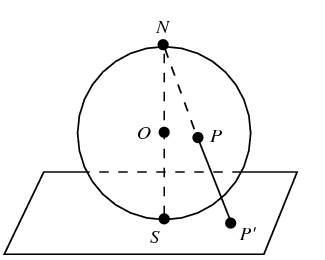Figure 2: The sphere is projected onto the plane by drawing a line from N through a point P, sending P to where the line crosses the plane (P’).

If we cut out some small, closed region from our sphere, or even a single point, then our new metric space (under the same definition of distance) ceases to be complete. Consider if a country, on Earth, closed itself to foreigners. I would never be able to travel to this country, despite the fact that the distance between me and its border would be finite. If we want to consider the Earth, without this country, to be a complete system, we must redefine distance so that the distance from anywhere on our space to the border of this country is infinite. To illustrate how we might define such a distance, let us look at a sphere which has a single point$N$ removed from it. We may transform any point of the once-punctured sphere onto the Euclidean ($\mathbb R^2$) plane via the continuous bijection known as the Stereographic Projection (Figure 2). We then say that the distance between two points on the sphere, p and q, is the Euclidean distance between p’ and q’. This metric, it turns out, is complete on the once- punctured sphere. Through a similar process, we may induce a metric on the a sphere with two distinct punctures using the Eucledian metric (we won’t go into detail about that process here).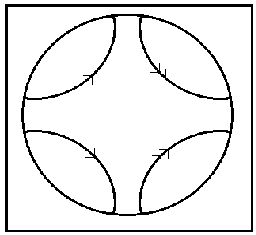Figure 3: Starting on the disk, we see that we can connect sides to make a pair of pants.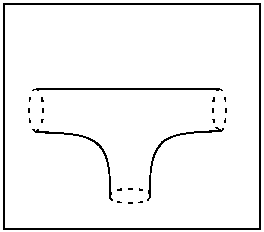Figure 4: The pair of pants can be deformed into a sphere with three holes.

So then, if we have a simple example of a metric on the sphere, and a way to induce a metric on the spheres with one or two punctures using Euclidean space, is it possible to find a complete  metric on the thrice-punctured sphere? The answer is yes… but not by relating our back to Euclidean space.

Consider the shape, S, formed by four arcs in a unit disk and its edge connecting them (Figure 3). Getting rid of the unnecessary portion of the disk, we may fold S over the x axis and connect the top two arcs to the bottom two. We then take any two points along these arcs sharing an x-coordinate and define them to be equivalent (”gluing” them together so to speak).  This gluing forms what we call a pair of pants. Note that this shape has three holes in it, suggesting a similarity to the thrice punctured sphere. In fact, we can stretch it so that it exactly resembles such a sphere (Figure 4). By making the arcs intersect the edge of the sphere closer to one another, we may shrink these punctures down to single points.

Recall how we defined a metric on the punctured sphere by sending points to the Euclidean plane. We now define distance on the thrice-punctured using the mapping from the sphere back into the disk. Note that, for any path on the sphere, we may unwrap the sphere back into$S$, giving us some collection of paths in the disk. We then define the length of a path by however long its segments are on the unwrapped space. The distance between two points, then, is the largest value which is shorter than the lengths of all these paths.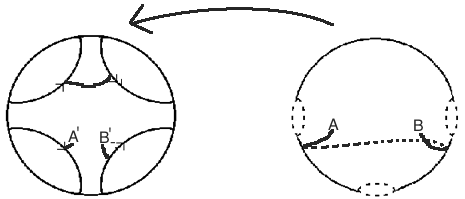Figure 5: Showing how a path on the thrice-punctured sphere can be “unwrapped” back onto our original shape S. The length of the path then, from A to B, is the length of each of the segments of its inverse image in S.

This just means that we need to clarify our notion of distance on$S$, inside of the open unit disk. Now, we could use the Euclidean metric again, but this would still fail to give us a complete space. If I take the sequence$0, \frac 12, \frac 23, \frac 34,\frac 45$…, it should not be hard to see that the sum of the distances between consecutive points is just one. Indeed, the first$n$ many pairs of distances will just be the difference between zero and the$n+1$th term. As the sequence goes to one, the sum of these lengths goes to one. However, the fact that the sequence converges to one also causes a problem, as one corresponds with one of the punctures on our sphere. So then, we cannot have a complete sense of distance on the open disk using the Euclidean sense of distance.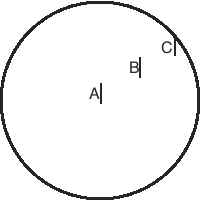Figure 6: Taking three line segments A,B, and C of equal Euclidean length, it turns out that the hyperbolic length of them is increased the closer they are to the boundary. The length of B is greater than that of A, while we say that the length of C is infinite, as it approaches the boundary.

This is where hyperbolic space comes in. On the unit disk model, we think of hyperbolic space as becoming squished near the boundary. Though the Euclidean distance between two points in the disk may only be some value d, if we slide them towards the edge of the disk (without changing their Euclidean displacement) then the hyperbolic distance between them approaches infinity. Though a severe over-simplification, think about taking a 6″ pen and holding it horizontally in front of your face. Though we might say that the pen has a constant Euclidean length, regardless of where you place it, if you slowly move the pen towards one of your eyes (while holding the other shut) the width of the pen appears to grow arbitrarily large, until it is wider than your field of vision.

It is this property that makes our space complete. Looking back at our previous example, of the sequence converging to one. In Euclidean space, the distances between consecutive terms added up to one. However, we now have that the magnitude of these distances gets amplified as they get closer to one. In fact, since the line segment from zero to one has infinite length in hyperbolic space, we infer that the sum of these distances (which are just the pieces of this line segment) must also grow arbitrarily large as we add them. We see then that this fixes the problem we had when we tried to apply a Euclidean manifold.

By repairing this issue, it turns out that our new space is, in fact, complete. The existence of a complete metric induced by the hyperbolic disk is implied by applying the Poincare’ Polygon Theorem to$S$. Some may wonder then, why am I making such a big deal about completeness? It turns out that many forms of advanced mathematics may only work to their full potential on complete spaces. My entire preoccupation with the thrice punctured sphere began when doing research for my honors thesis in Complex Analysis. Despite being a completely different field, I needed this definition of distance. The Schwarz–Ahlfors–Pick Theorem directly relates this metric to the idea of differentiability, allowing us to create differentiable functions on the open disk to this punctured sphere which don’t increase hyperbolic distance.

This entry was posted in Uncategorized and tagged , . Bookmark the permalink.